# AP Calculus Review: Properties of Exponents and Logarithms

To do well on the AP Calculus exam, it is important to have a strong algebra background. When you get to the AP exam, you don’t want to be worrying about some of the subtleties of manipulating equations. Rather, you want to be focusing on the questions at hand. One of the most common areas students make mistakes are with the exponents and logarithms, which are very important both when taking derivatives and when integrating equations.

## Important exponent and logarithm rules for AP Calculus

Below is a list of exponent and logarithm rules with which you should be familiar.

### Exponent Rules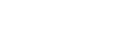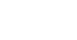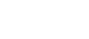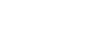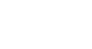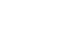## Exponent and logarithm practice problems

Some common questions on the AP Calculus exam involve exponential growth and decay. The growth problems often come up in the form of interest rate questions, where the growth problems often appear in the form of half-life questions. You should be comfortable coming up with an equation to model the following three scenarios (answers below:

a) A bank gives interest at a rate of 4% per year. If the interest in compounded quarterly and one begins with \$200, write a formula that will give the total balance in an account as a function of time.

b) In the above question, interest was compounded continuously. Write a formula that will give the total balance as a function of time.

c) If the half-life of carbon-14 is 5730 years, write a formula that will give the total percentage of an initial amount remaining as a function of time.

Below is a list of problems that you can use to practice your algebra before the AP Exam. They involve the above exponent rules, logarithm rules, and quickly changing between bases.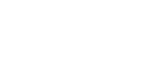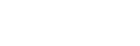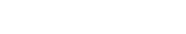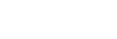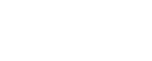## Exponent and logarithm practice problems solutions

### Scenarios

a) T = 200*(1+.04/4)4t

b) T= 200(e).04t

c) Percent = (1/2)t/5730

### Algebra Practicex = 10/3x = -2x = 6/17x = 7/2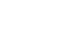x = 6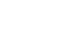x = 3/2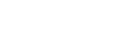x = 0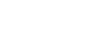x = 2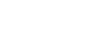x =0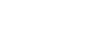x = 9/3x = 0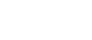You should be able to solve logarithm and exponential questions quickly on a calculator. Remember, when you see a log function on your calculator, it is assumed to be base 10, unless otherwise specified. Some calculators (including most graphing calculators) are able to solve log functions which do not have a base 10, but this is not all models. If your calculator cannot do this, or if it is an option buried behind menus and difficult to get to (which is also the case in many graphing calculators), there is a short cut to answering these problems quickly: the change of base formula or…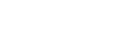This means that if we are looking for log510, we can plug into our calculator (log 10)/(log 5) and get the correct answer.

Being fluent with the algebra we learned before calculus, including little tricks like the change of base formula, can save us a lot of time on the AP Exam and allow us time to focus on the more difficult parts of the test.

#### Improve your SAT or ACT score, guaranteed. Start your 1 Week Free Trial of Magoosh SAT Prep or your 1 Week Free Trial of Magoosh ACT Prep today!## Author

•Zachary is a former mechanical engineer and current high school physics, math, and computer science teacher. He graduated from McGill University in 2011 and spent time in the automotive industry in Detroit before moving into education. He has been teaching and tutoring for the past five years, but you can also find him adventuring, reading, rock climbing, and traveling whenever the opportunity arises.